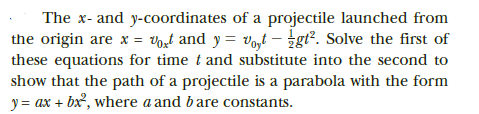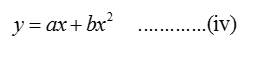# The x- and y-coordinates of a projectile launched fromthe origin are x = Vort and y = voyt – ¿gt². Solve the first ofthese equations for time t and substitute into the second toshow that the path of a projectile is a parabola with the formy = ax + bx, where a and bare constants.

Question
10 viewshelp_outlineImage TranscriptioncloseThe x- and y-coordinates of a projectile launched from the origin are x = Vort and y = voyt – ¿gt². Solve the first of these equations for time t and substitute into the second to show that the path of a projectile is a parabola with the form y = ax + bx, where a and bare constants. fullscreen
check_circle

Step 1

Given data:

Step 2

Plugging in the value of t in equation (i):

Step 3

The equation of the parabola:...

### Want to see the full answer?

See Solution

#### Want to see this answer and more?

Solutions are written by subject experts who are available 24/7. Questions are typically answered within 1 hour.*

See Solution
*Response times may vary by subject and question.
Tagged in

### Other